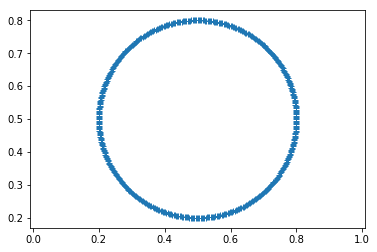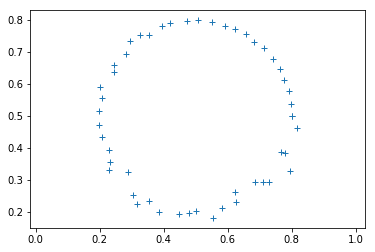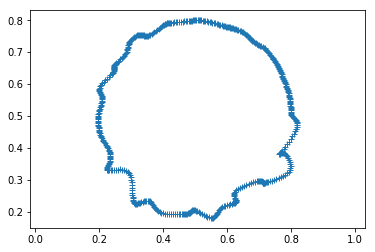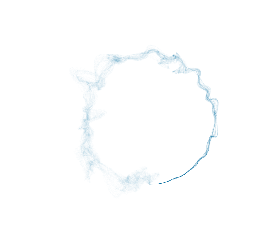# Splinart on a circle¶

In this tutorial, we will see how to use splinart with a circle.

First of all, we have to create a circle.

In :

import splinart as spl
center = [.5, .5]


In the previous code, we create a discretization of a circle centered in $$[0.5, 0.5]$$ with a radius of $$0.3$$. We don’t specify the number of discretization points. The default is 30 points.

We can plot the points using matplotlib.

In :

%matplotlib inline

In :

import matplotlib.pyplot as plt
plt.axis("equal")
plt.plot(path[:, 0], path[:, 1], '+')

Out:

[<matplotlib.lines.Line2D at 0x7fc91dfe6048>]## The sample¶

In order to compute a sample on a given cubic spline equation, we need to provide a Python function that gives us the x coordinates. We can choose for example.

In :

import numpy as np
def x_func():
nsamples = 500
return (np.random.random() + 2 * np.pi * np.linspace(0, 1, nsamples))%(2*np.pi)


We can see that the points are chosen between $$[0, 2\pi]$$ in a random fashion.

## The cubic spline¶

Given a path, we can apply the spline function in order to compute the second derivative of this cubic spline.

In :

yder2 = spl.spline.spline(theta, path)


And apply the equation to the sample

In :

xsample = x_func()
ysample = np.zeros((xsample.size, 2))
spl.spline.splint(theta, path, yder2, xsample, ysample)


which gives

In :

import matplotlib.pyplot as plt
plt.axis("equal")
plt.plot(ysample[:, 0], ysample[:, 1], '+')

Out:

[<matplotlib.lines.Line2D at 0x7fc91e05feb8>]We can see the sample is well defined around the circle that we defined previously.

Now, assume that we move randomly the points of the circle with a small distance.

In :

spl.compute.update_path(path, scale_value=.001, periodic=True)

In :

import matplotlib.pyplot as plt
plt.axis("equal")
plt.plot(path[:, 0], path[:, 1], '+')

Out:

[<matplotlib.lines.Line2D at 0x7fc91da92e10>]And we compute again the sample of the new cubic spline equation.

In :

yder2 = spl.spline.spline(theta, path)
spl.spline.splint(theta, path, yder2, xsample, ysample)
spl.compute.update_path(path, scale_value=.001, periodic=True)

In :

import matplotlib.pyplot as plt
plt.axis("equal")
plt.plot(ysample[:, 0], ysample[:, 1], '+')

Out:

[<matplotlib.lines.Line2D at 0x7fc91d94f748>]The circle is deformed.

This is exactly how works splinart. We give a shape and at each step

• we perturb the points of this shape
• we compute a sample an this new cubic spline equation
• we add the pixel with a given color on the output image

And we do that several time. We can have the following result

In :

img_size, channels = 1000, 4
img = np.ones((img_size, img_size, channels), dtype=np.float32)


In :

spl.show_img(img)